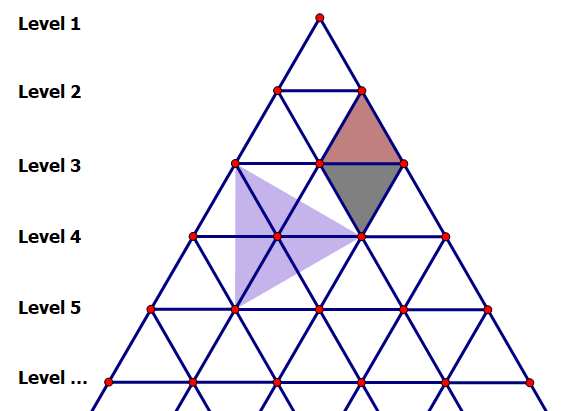시간 제한 메모리 제한 제출 정답 맞은 사람 정답 비율
2 초 512 MB 2 2 2 100.000%

## 문제

A side surface of a pyramid can be cut into many equilateral triangles, whose vertices can be grouped into different levels from top to bottom, such that the first level contains one vertex, the second level contains two, and so on.As the image shows, for example, two adjacent level-k vertices with a level-(k − 1) vertex can form an upright equilateral triangle, and two adjacent level-k vertices with a level-(k + 1) vertex can form an inverted equilateral triangle as well. Also, three vertices at three different levels can form an equilateral triangle, which may be oblique.

If we only consider vertices between level l and level r (inclusive), in how many ways can we choose three equidistant vertices so that they can form an equilateral triangle?

## 입력

The input contains several test cases. The first line contains an integer T indicating the number of test cases. The following describes all test cases. For each test case:

The only line contains two integers l and r.

## 출력

For each test case, output a line containing “Case #x: y” (without quotes), where x is the test case number starting from 1, and y is the answer to this test case.

## 제한

• 1 ≤ T ≤ 3 × 105
• 1 ≤ l ≤ r ≤ 105

## 예제 입력 1

3
1 3
2 4
3 5


## 예제 출력 1

Case #1: 5
Case #2: 12
Case #3: 20